Note that these animations also show the behaviour of a string.

The first harmonic (also known as the fundamental) can be seen in Figure #1 below. The top plot with the red line is the graph typically seen in textbooks. It shows, in any frame, the instantaneous displacement of the air particles in the closed pipe (shown as circles in the lower plot) relative to their "resting" position. When the red line is positive (higher), the air particles have moved to the right. When the red line is negative (lower) the air particles have moved to the left.

Notice that the string (the red line) is clamped to an immovable object on each end (therefore it has two "high impedance terminations"). Similarly, the caps on the ends of the pipe are high impedance. This means that the ends of the string can't move, and neither can the last air particles at the ends of the pipe.

Notice as well that the resonance has a wavelength equal to two times the length of the pipe. This is more easily seen in the red-lined plot than the drawings of the particles in the pipe.

One important thing to note here is that, typically, in textbooks, you'll see a plot that shows either the pressure or the velocity of the air particles. The red lines shown here are neither of these. As I mentioned above, they're the displacement of the air particles.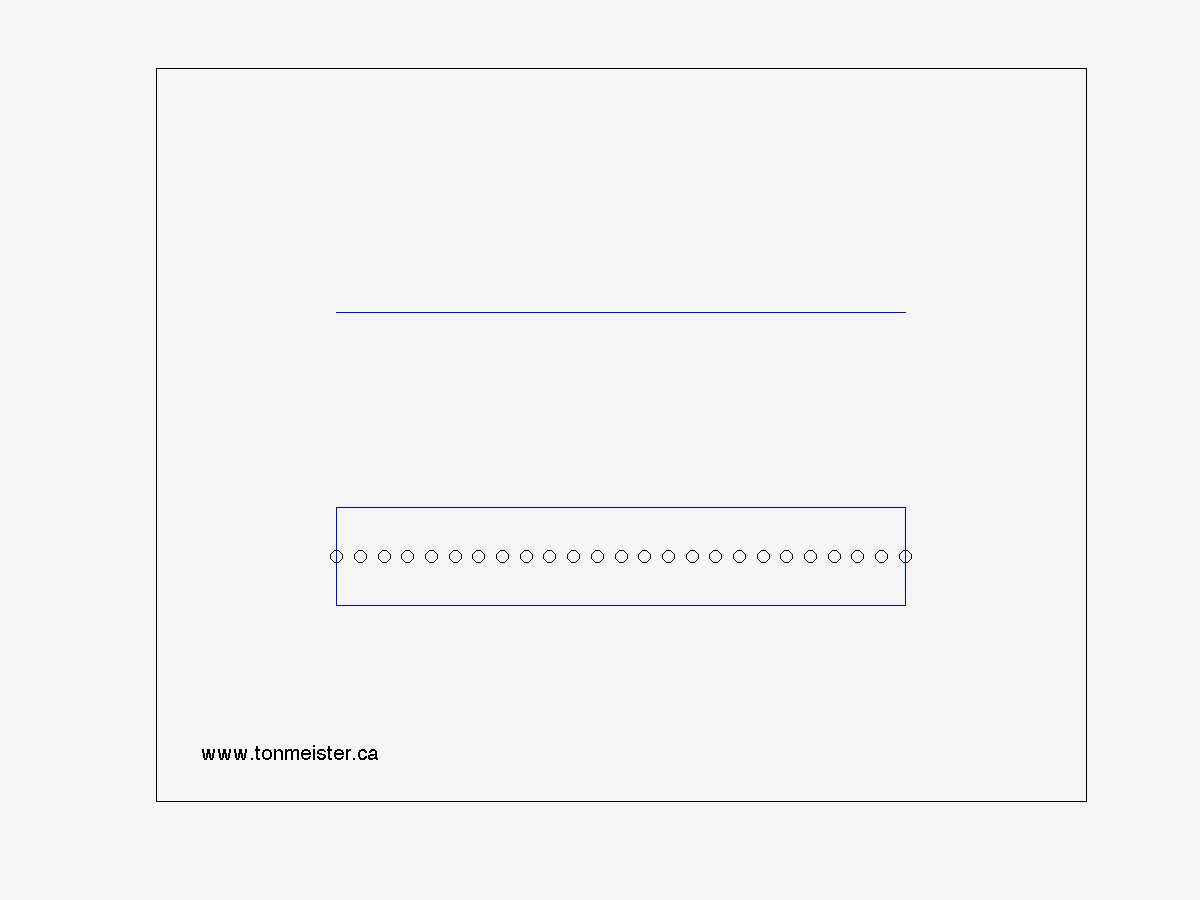Figure 1 - Harmonic #1

The second harmonic (shown below) occurs when the wavelength of the resonance equals the length of the pipe. This means that we have a point at the centre of the pipe where the air particles (and the string) don't move.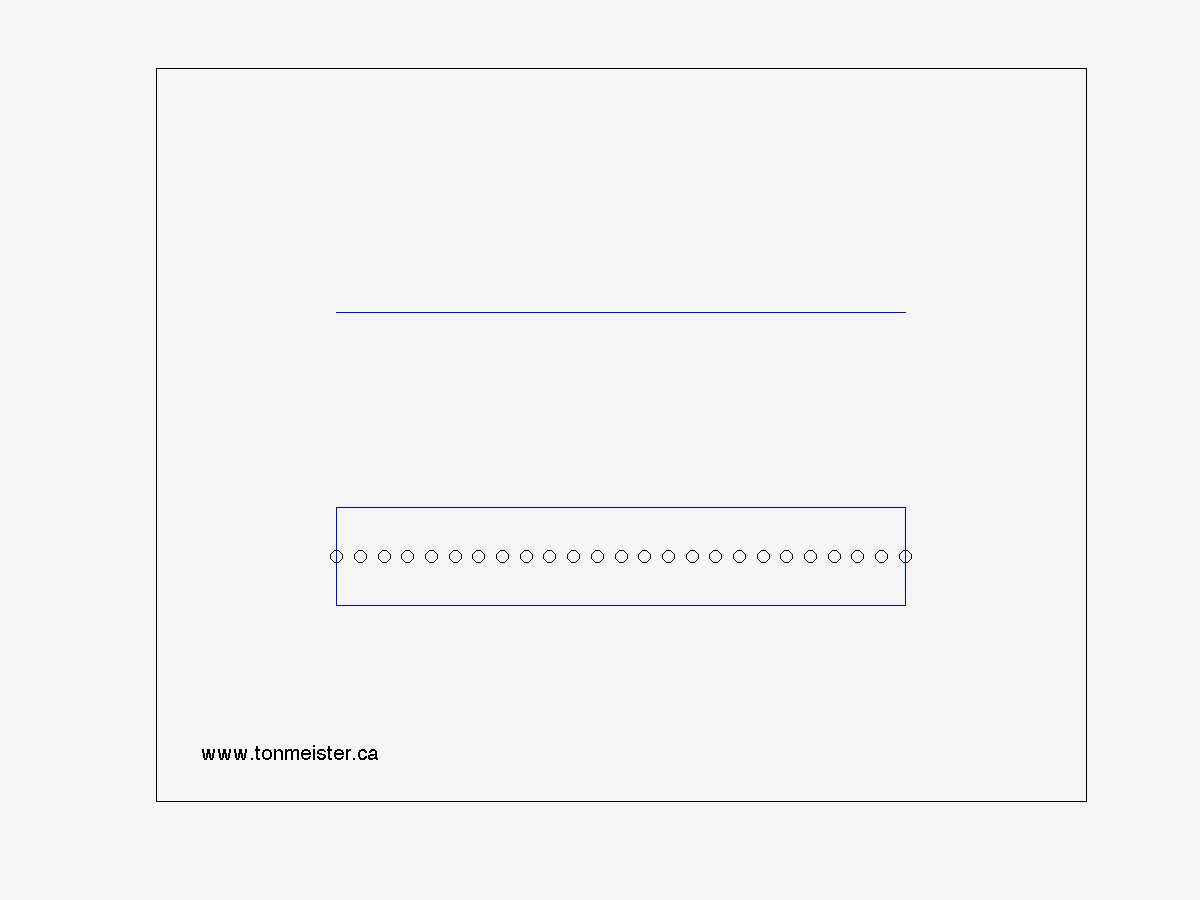Figure 2 - Harmonic #2

The third harmonic (shown below) occurs when the wavelength of the resonance equals 1.5 times the length of the pipe. This means that we have two points at the centre of the pipe where the air particles (and the string) don't move.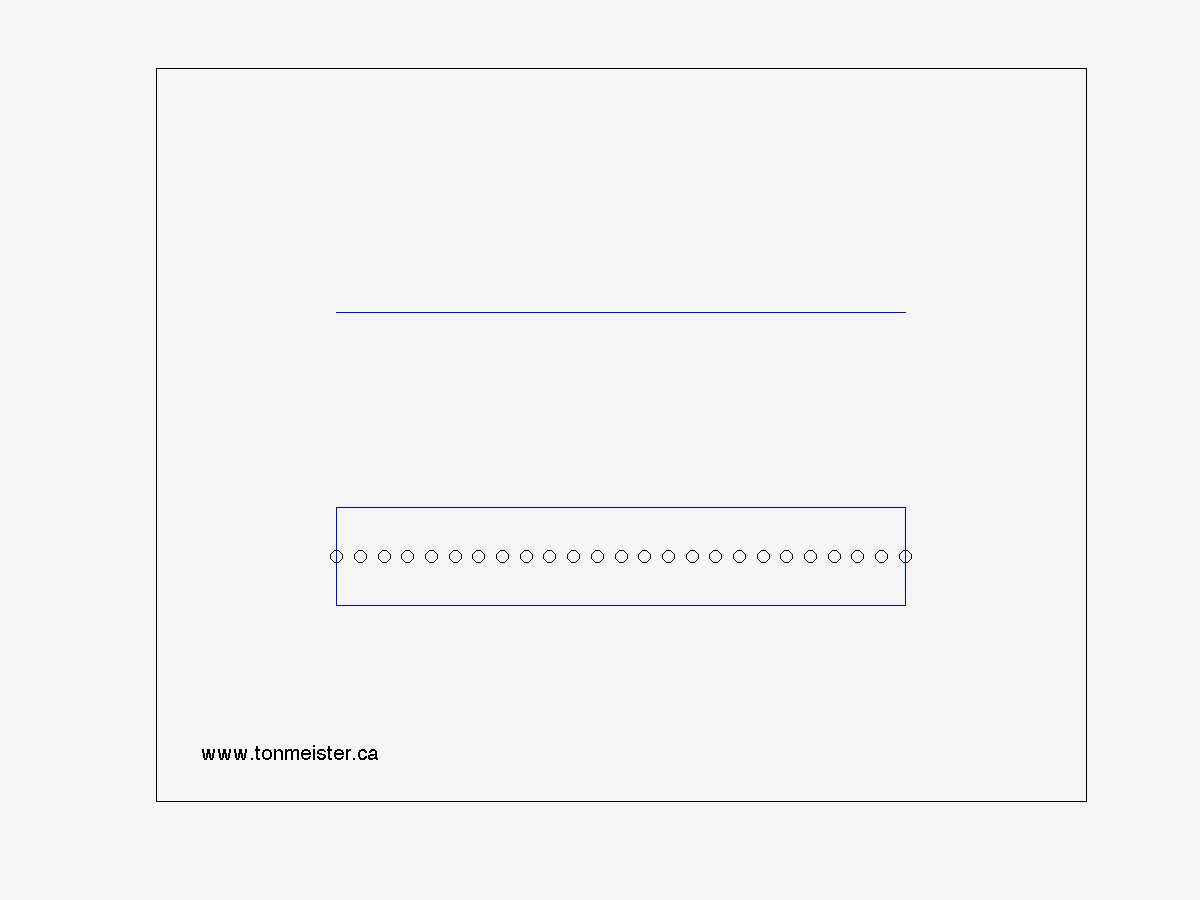Figure 3 - Harmonic #3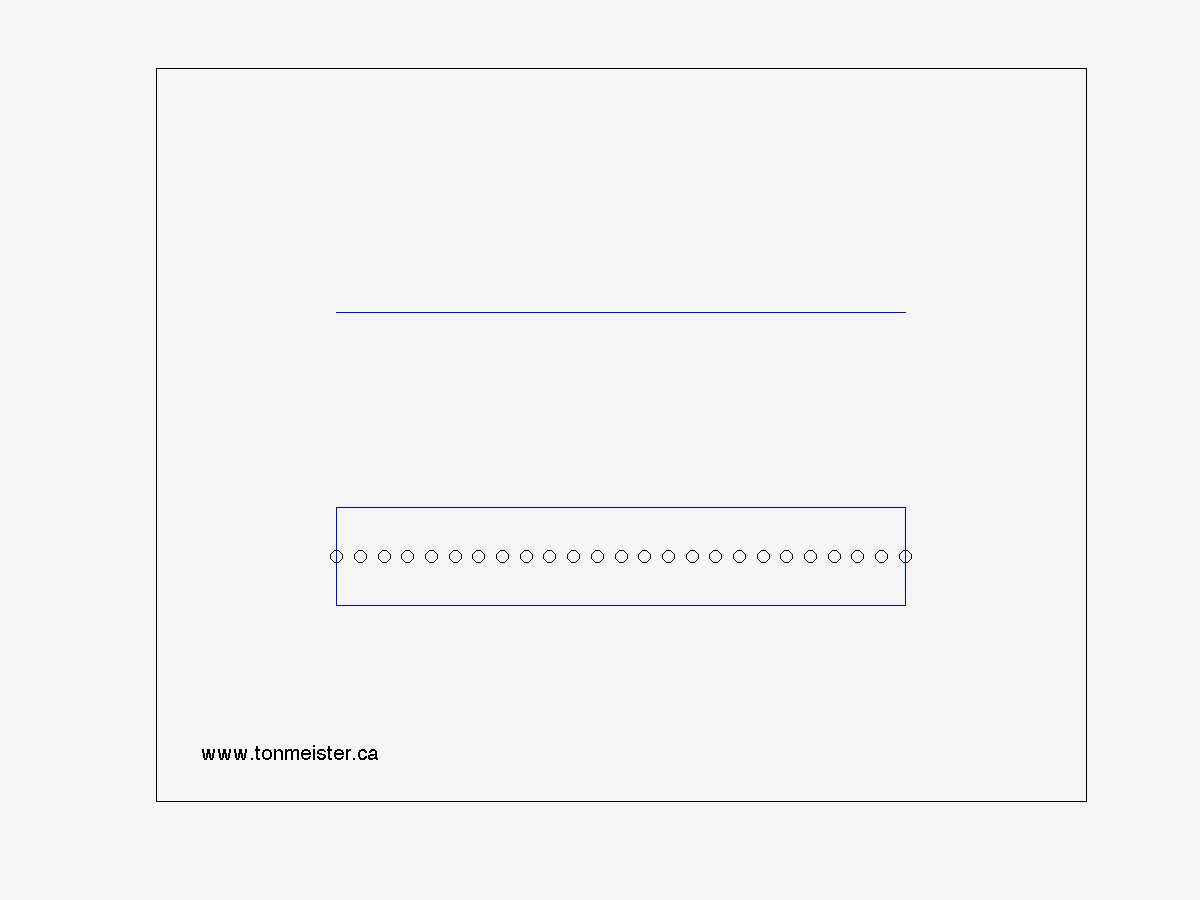Figure 4 - Harmonic #4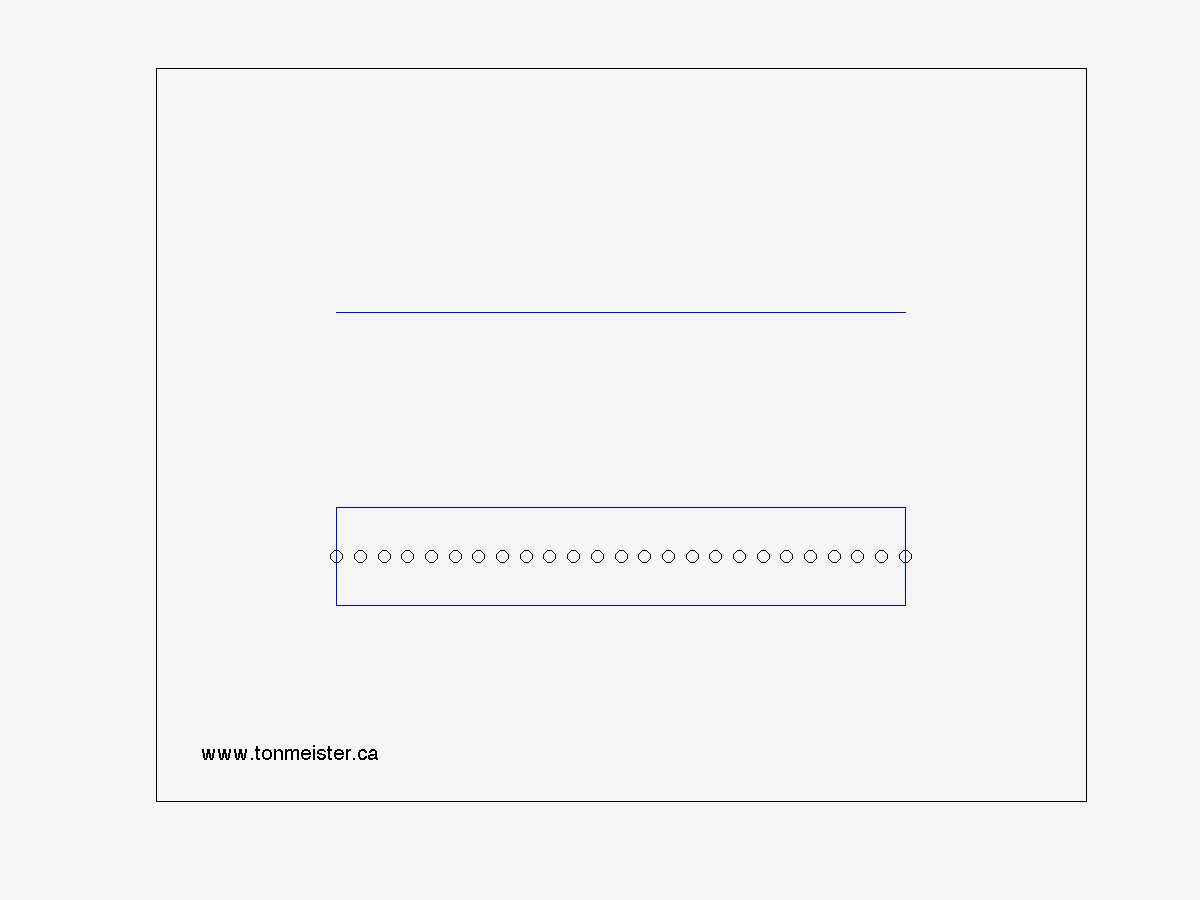Figure 5 - Harmonic #5